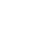Kilowatts to Amperes Calculator for AC Motors

3
2740Last Updated on January 18, 2022 by Krunal Shah (Mod)

This calculator is to find out input current of induction motor. You can find amperes of both single phase and three phase induction motor when kW is known to you.

 .specialclass lblTest { margin-left: 200px; }

How to use the calculator?

• From the drop down, select the phase.
• For this calculator, note down the voltage, efficiency, power factor and kW of the motor. You can find details from the motor nameplate.
• Enter the data collected in respective fields.
• You can take value 0.8 for pf and 0.8 for efficiency if it is not available on motor name plate.
• The calculator would show automatically the calculated value.

Formulas for kW to Amperes calculation

Single Phase

For single phase, amperes (I) is equal to kilowatts (kW) multiply by 1000 whole divided by Voltage (V) multiply by Power Factor (PF) multiply by Efficiency (EFF). Multiplying by thousand would convert kilowatts into watts.Example: Let us say you have 50 kW motor with rated voltage 230 VAC, power factor 0.80 and efficiency 0.85, then the input current of motor is 319.693 amps.

Three Phase

For three phase, amperes (I) is equal to kilowatts (kW) multiply by 1000 whole divided by Voltage (V) multiply by Power Factor (PF) multiply by Efficiency (EFF) multiply by square root of 3.Example: Let us say we have 50 kW motor with rated voltage as 415 VAC, power factor as 0.80 and efficiency as 0.85, then the input current of motor is 102.416 amps.

1.ปั้มไลค์

Like!! Great article post.Really thank you! Really Cool.

•Krunal Shah

Thank you

2.pavan kumar M.tech